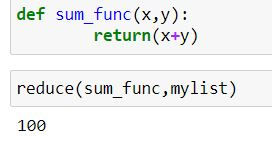top of page
Search
• Ekta Aggarwal

# Reduce and accumulate

Updated: Jan 26, 2021

To find cumulative sum or products or to compare elements in a list sequentially, reduce( ) function in Python can be your saviour!

It belongs to library functools so we need to import it first.

`from functools import reduce`

Let us import another library operator which will be used during this tutorial.

`import operator`

Let us create our list:

`mylist = [10,20,30,40]`

Syntax:

reduce (function , list, initial_value <optional>)

### Finding cumulative sum:

To find cumulative sum for our data we shall use add( ) function from library: operator

`reduce(operator.add, mylist)`How is it working?

reduce function firstly sums first two elements of our list: 10 and 20 and stores the resultant output i.e. '30' in memory.

Next, it adds the next element i.e. 30 to our stored output, thus it stores the output 60 in memory.

Finally it takes the fourth element from the list i.e. 40 and adds it to 60, so 100 is our output!

You can also implement a lambda function in the first argument.

`reduce(lambda x,y:x+y,mylist)`Moreover, you can also create a UDF to get sum of 2 numbers and then pass on the UDF to reduce( ) function.

```def sum_func(x,y):
return(x+y)

reduce(sum_func,mylist)```### Initializer in reduce:

In reduce( ) function you can also set an initialized value that is it will start the calculations starting from that point

In the below code our initial value is 20, and thus the computations in reduce( ) function begin using 20, hence the output is 120 (not 100)

`reduce(lambda x,y:x+y,mylist,20)`### Finding cumulative product:

Using the following lambda function( ) we can calculate the cumulative product of all the elements of the list.

`reduce(lambda x,y:x*y,mylist)`

Alternatively, you can use mul function from operator library to multiply the elements:

`reduce(operator.mul, mylist)`

Task: Initialize the product from 10:

`reduce(operator.mul, mylist,10)`### If else in reduce function:

Task: To find the largest element from our list:

In the following code we are using list comprehension: which is as follows:

value_if_true condition_to_be_evaluated else value_if_condition_is_false

`reduce(lambda a,b : a if a > b else b,mylist)`

Above code compares first two elements of the list and stores the maximum element (i.e. 20) in the memory, then Python compares third element (30) with the 20 and stores maximum out of these (i.e. 30) in the memory. Finally it compares the last element (40 ) with 30 and gives 40 as final output!### accumulate( ) function

There is a similar function in Python i.e. accumulate( ) function in itertools library which also does the calculations sequentially like reduce( )

Syntax:

accumulate(list, function)

Difference between reduce and accumulate:

• Reduce( ) returns the final result as the output while accumulate( ) returns all of the intermediate calculations.

• Output of reduce( ) is a scalar, while accumulate( ) returns a list.

• The last element of accumulate( ) is the value equal to the scalar given by reduce( )

Let us firstly load the library to use accumulate:

`import itertools`

Comparing the output using accumulate and reduce ( )

```list(itertools.accumulate(mylist,operator.mul))

reduce(operator.mul,mylist)```bottom of page